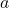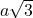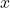Which solution finds the value of x in the triangle below? A right triangle is shown. The hypotenuse has a length of 8. Another

Question

Which solution finds the value of x in the triangle below?

A right triangle is shown. The hypotenuse has a length of 8. Another side has a length of x. The angle between the hypotenuse and the other side is 60 degrees.

in progress 0
5 months 2021-08-11T11:08:36+00:00 2 Answers 9 views 0

4

Step-by-step explanation:

Since this is a right triangle, and one of the angles measures 60 degrees, we can conclude that the last side measures 30 degrees.

We can see that this is a 30-60-90 degree triangle.

The rules of 30-60-90 degree triangles are that the side opposite the 90 degree angle, or the hypotenuse can be measured with the variable. The side opposite the 30 degree angle can be measured with, and the side opposite the 60 degree angle will be measured with.

We can see that 8 representsbecause it is the hypotenuse. Since the side markedis separated by the hypotenuse by an angle of 60 degrees, we note that side markedis opposite the angle measuring 30 degrees. We note that the side opposite 30 degrees is marked, and since we already know that 8 is equal to, we realize that the side marked x is equal to, or 4.

2. I didn’t get it can you post a picture with it too?